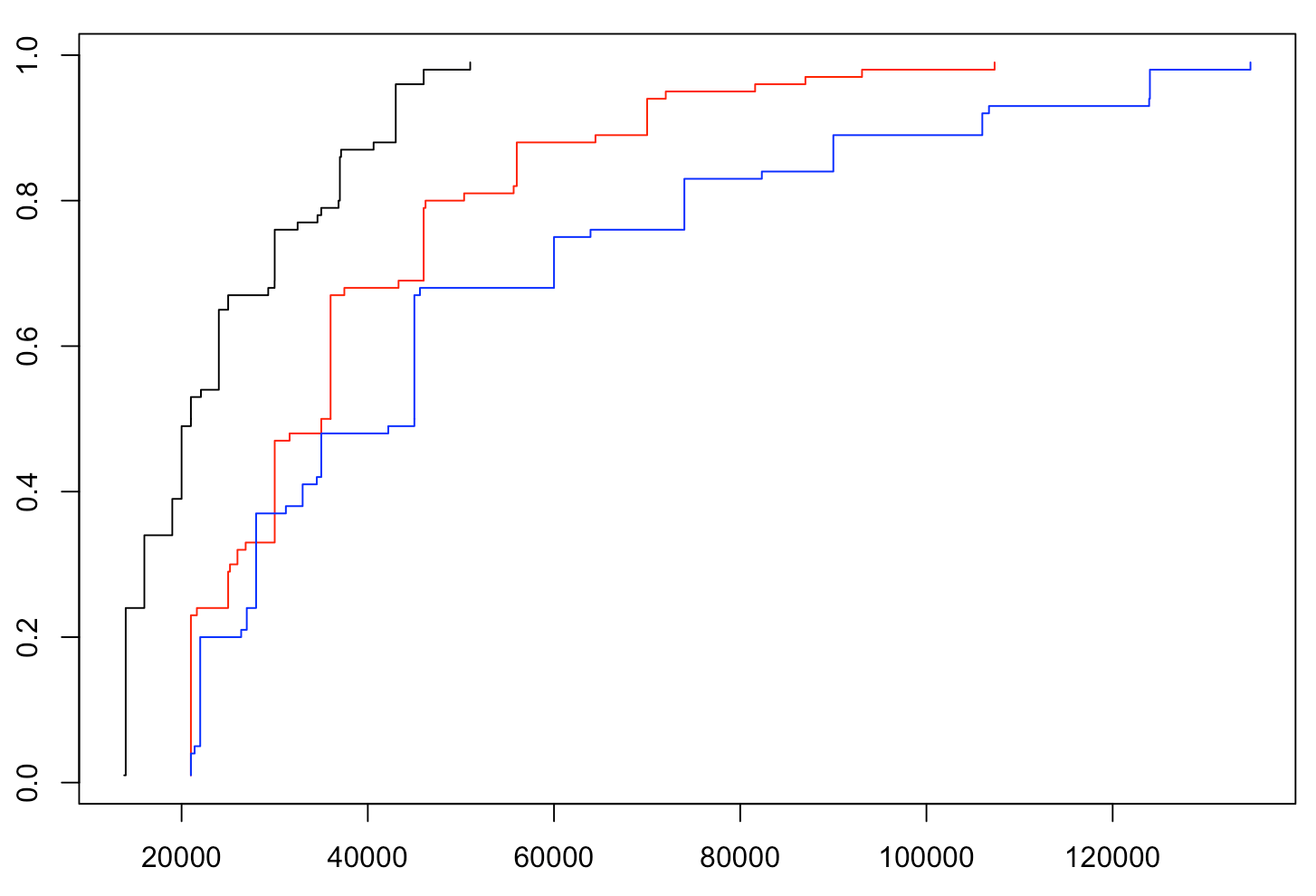Want to share your content on R-bloggers? click here if you have a blog, or here if you don't.

In 2012, Danielle Herbert, Adrian Barnett, Philip Clarke and Nicholas Graves published an article entitled “on the time spent preparing grant proposals: an observational study of Australian researchers“, whose conclusions had been included in Nature under a more explicit title, “Australia’s grant system wastes time” ! In this study, they included 3700 grant applications sent to the National Health and Medical Research Council, and showed that each application represented 37 working days: “Extrapolating this to all 3,727 submitted proposals gives an estimated 550 working years of researchers’ time (95% confidence interval, 513-589)“. But in these times when I have to write my funding application, I find that losing 37 days of work is huge. Because it’s become the norm! And somehow, it’s sad.Forget about the crazy idea that I would rather, in fact, spend more time doing my research. In fact, the thought I had this morning was that it is rather sad that in the Faculty of Science, mathematicians are asked to spend a considerable amount of time, comparable to that required of physicists or chemists, for often smaller amounts of funding… And I thought it could be easily verified. We start by retrieving the discipline codes

```url="http://www.nserc-crsng.gc.ca/NSERC-CRSNG/FundingDecisions-DecisionsFinancement/ResearchGrants-SubventionsDeRecherche/ResultsGSC-ResultatsCSS_eng.asp"
library(XML)
GSC=tables[]\$V1
GSC=as.character(GSC[-(1:2)])
namesGSC=tables[]\$V2
namesGSC=as.character(namesGSC[-(1:2)])```

We’re going to need a small function, to remove the \$ and other symbols that pollute the data (and prevent them from being treated as numbers)

```library(stringr)
Correction = function(x) as.numeric(gsub('[\$,]', '', x))```

We will now read the 12 pages, and harvest (we will just take the 2017 data, but we could go back a few years before)

```grants= function(gsc){
url=paste("http://www.nserc-crsng.gc.ca/NSERC-CRSNG/FundingDecisions-DecisionsFinancement/ResearchGrants-SubventionsDeRecherche/ResultsGSCDetail-ResultatsCSSDetails_eng.asp?Year=2017&GSC=",gsc,sep="")
library(XML)
X=as.character(tables[]\$"Awarded Amount")
A=as.numeric(Vectorize(Correction)(X))
return(c(median(A),mean(A),as.numeric(quantile(A,(1:99)/100))))
}
M=Vectorize(grants)(GSC[1:12])```

The average amounts of individual grants can be compared,

`barplot(M[2,])`In mathematics, the average grant amount is \$24400. If we normalize by this quantity, we obtain

`barplot(M[2,]/M[2,8])`In other words, the average amount of a (individual) grant in chemistry (to pay for students, conferences, etc.) is twice that in mathematics, 60% higher in physics than in maths…

We can also look at the median values (rather than the averages)

`barplot(M[1,])`Here again, it is in mathematics that it is the weakest….

`barplot(M[1,]/M[1,8])`in comparable proportions. If we think that the time spent writing should be proportional to the amount allocated, we should spend half as much time in math as in chemistry.

Cumulative functions can also be ploted,

```plot(M[3:101,8],(1:99)/100,type="s",xlim=range(M))
lines(M[3:101,5],(1:99)/100,type="s",col="red")
lines(M[3:101,4],(1:99)/100,type="s",col="blue")```with math in black, physics in red, and chemistry in blue. What is surprising is the bottom part: a “bad” researcher in chemistry or physics will earn more than the median researcher in mathematics…

Now that my intuition is confirmed, I have to go back, writing my proposal… and explain to my coauthors that I have to postpone some research projects because, well, you know…